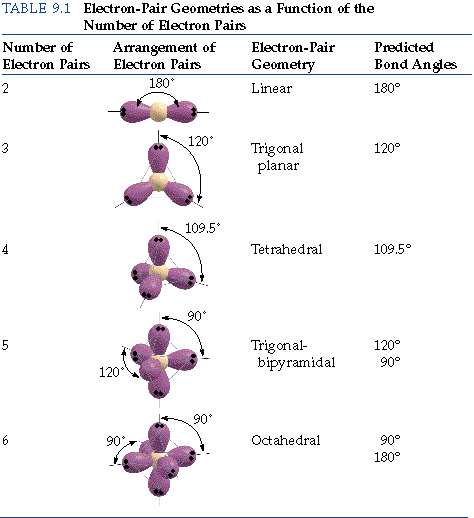# How do you draw VSEPR diagrams?

Jan 1, 2014

The Valence Shell Electron Pair Repulsion Theory (VSEPR) helps us to understand the 3D structure of molecules.

#### Explanation:

The general concept is that the pairs of electrons repel each other and try to locate themselves as far as possible from each other about a given nucleus.

Hence, for two pairs of electrons on a nucleus, the two pairs would locate themselves exactly opposite each other, forming a bond angle of exactly 180°.

If three pairs exist, they will locate themselves in a plane about the nucleus at angles of 120° from each other.

Here is a table of the electron pair geometries as a function of the number of electron pairs.To determine the shape of ${\text{SO}}_{2}$, for example, we first determine the Lewis dot structure of ${\text{SO}}_{2}$.The central atom, $\text{S}$, has three groups bonded to it, two oxygen atoms and a lone pair.

The electron pair geometry of ${\text{SO}}_{2}$ is trigonal planar. It is drawn asThe molecular geometry of ${\text{SO}}_{2}$ is not trigonal planar.

In determining the molecular shape, we consider only the positions of the atoms, not the lone pairs.

So, the molecular shape of ${\text{SO}}_{2}$ is bent and is represented as:The lone pair of electrons occupies a relatively large volume, since they are held by only one atom.

They compress the bond angle between the oxygens and sulfur to less than 120°. The actual $\text{O-S-O}$ bond angle is 119.5°.

Here is a table listing the molecular shapes that correspond to various combinations of bonding pairs and lone pairs.